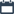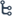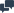Welcome to Instructbd

## Wednesday, 13 July 2016

•23:01:00
•••1 comment

## [Solution] Elementary Differential Equations and Boundary Value Problems by Boyce & Diprima - 9th Edition

#### About Solution Book:

Title: Elementary Differential Equations and Boundary Value Problems
Author : by William E. Boyce, Richard C. DiPrima
Edition: 9th
Total Page: 288

Size : 2 MB

#### Content:

• Introduction
• First Order Differential Equations
• Second Order Linear Equations
• Higher Order Linear Equations
• Series Solutions of Second Order Linear Equations
• The Laplace Transform
• Systems of First Order Linear Equations
• Numerical Methods
• Nonlinear Differential Equations and Stability
• Partial Differential Equations and Fourier Series
• Boundary Value Problems and Sturm–Liouville Theory
• Index

(you can also read this book online)

•22:34:00
•••1 comment

## [Book] Elementary Differential Equations and Boundary Value Problems by William E. Boyce, Richard C. DiPrima

#### This book is based on ordinary and Partial differential equations and boundary value problems. This book is primarily designed for undergraduate students of mathematics, science and engineering, who typically take a course on differential equations during their first or second year of study. The main prerequisite for reading the book is a working knowledge of calculus, gained from a normal two or three semester course sequence or its equivalent. Some familiarity with matrices will also be helpful in the chapters on systems of differential equations.  With respect to content, we provide this flexibility by making sure that, so far as possible, individual chapters are independent of each other. Thus, after the basic parts of the first three chapters are completed (roughly Sections 1.1 through 1.3, 2.1 through 2.5, and 3.1 through 3.5), the selection of additional topics, and the order and depth in which they are covered, is at the discretion of the instructor. Chapters 4 through 11 are essentially independent of each other, except that Chapter 7 should precede Chapter 9 and that Chapter 10 should precede Chapter 11. This means that there are multiple pathways through the book and many different combinations have been used effectively with earlier editions.

Title: Elementary Differential Equations and Boundary Value Problems
Author : by William E. Boyce, Richard C. DiPrima
Edition: 9th
Total Page: 738
Size : 4 MB

#### Content:

• Introduction
• First Order Differential Equations
• Second Order Linear Equations
• Higher Order Linear Equations
• Series Solutions of Second Order Linear Equations
• The Laplace Transform
• Systems of First Order Linear Equations
• Numerical Methods
• Nonlinear Differential Equations and Stability
• Partial Differential Equations and Fourier Series
• Boundary Value Problems and Sturm–Liouville Theory
• Index

(you can also read this book online)